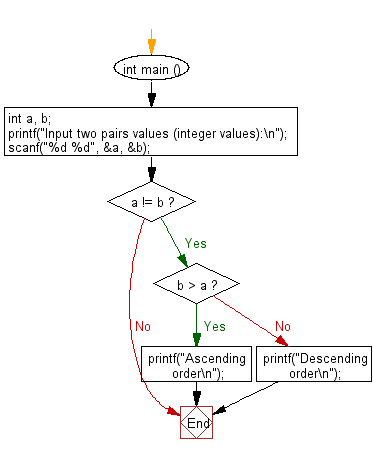﻿ C : From a pair of numbers, find ASC or DESC order

# C Exercises: Find Ascending or Descending order from a pair of numbers

## C Basic Declarations and Expressions: Exercise-116 with Solution

Write a C program that accepts a pair of numbers from the user and prints "Ascending order" if the two numbers are in ascending order, otherwise prints, "Descending order".

Sample Solution:

C Code:

``````#include <stdio.h>

int main () {
int a, b;

// Prompt user for input
printf("Input two pairs values (integer values):\n");

// Read two integer values from user and store them in 'a' and 'b'
scanf("%d %d", &a, &b);

// Check if 'a' is not equal to 'b'
if  (a != b) {

// Check if 'b' is greater than 'a'
if (b > a) {
printf("Ascending order\n"); // Print message for ascending order
}
else {
printf("Descending order\n"); // Print message for descending order
}
}

return 0; // End of program
}
``````

Sample Output:

```Input two pairs values (integer values):
12
35
Ascending order
```

Sample Output:

```Input two pairs values (integer values):
65
25
Descending order
```

Flowchart:C programming Code Editor:

What is the difficulty level of this exercise?

Test your Programming skills with w3resource's quiz.

﻿